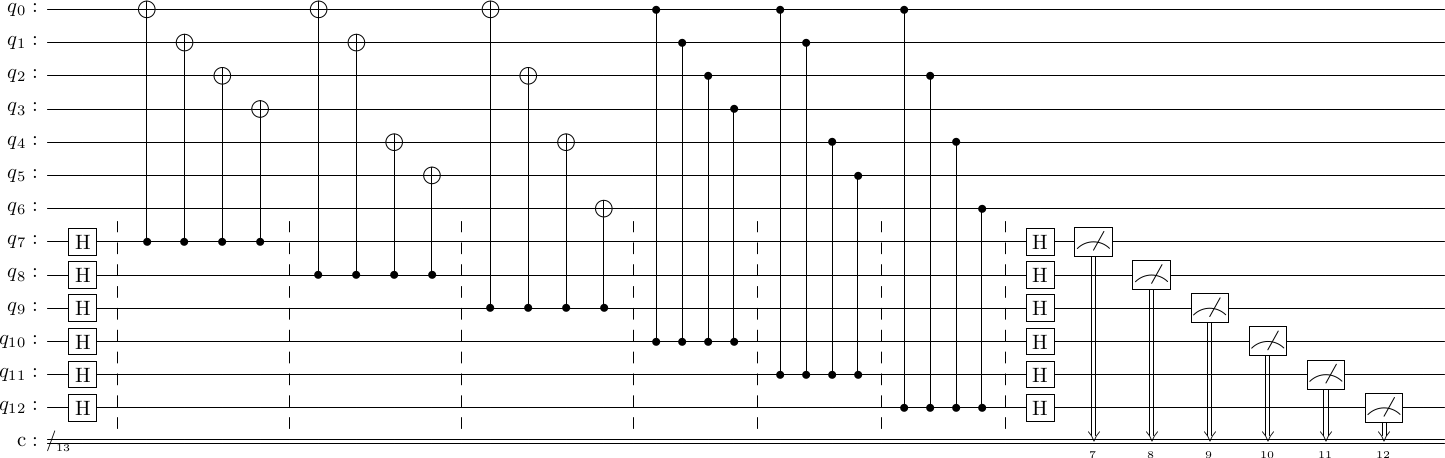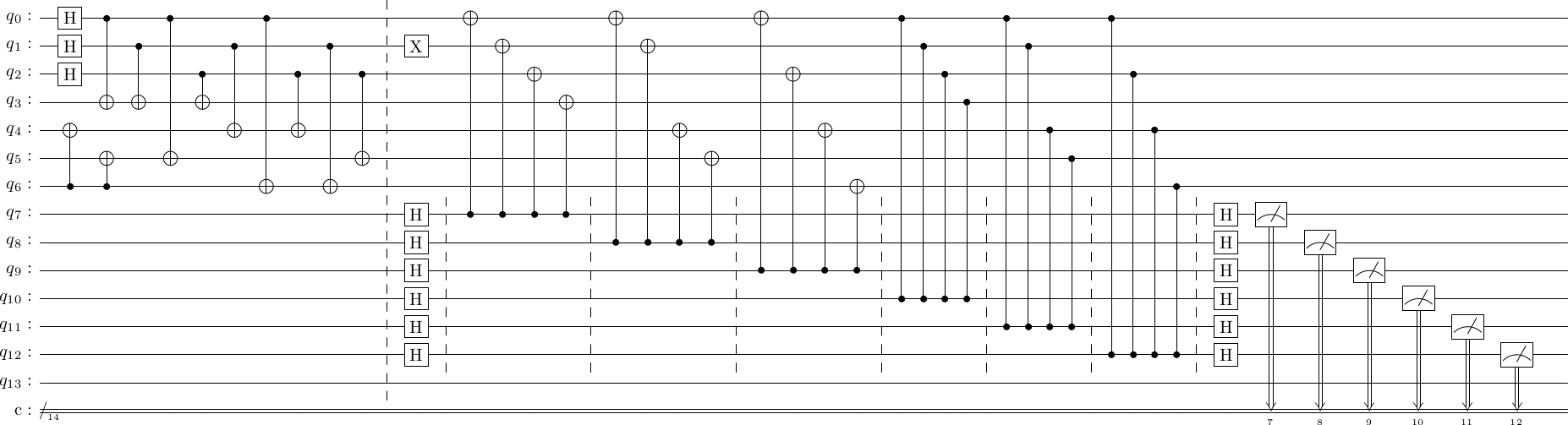# Syndrome measurements for stabilizer codes#

We will revisit syndrome measurements now, and demonstrate a general algorithm to construct them for stabilizer codes.

Our working example will be the Steane code.

import stac
cd = stac.CommonCodes.generate_code("[[7,1,3]]")
stac.print_matrix(cd.generator_matrix, augmented=True)
$\displaystyle \left(\begin{array}{ccccccc|ccccccc} 1 & 1 & 1 & 1 & 0 & 0 & 0 & 0 & 0 & 0 & 0 & 0 & 0 & 0 \\ 1 & 1 & 0 & 0 & 1 & 1 & 0 & 0 & 0 & 0 & 0 & 0 & 0 & 0 \\ 1 & 0 & 1 & 0 & 1 & 0 & 1 & 0 & 0 & 0 & 0 & 0 & 0 & 0 \\ 0 & 0 & 0 & 0 & 0 & 0 & 0 & 1 & 1 & 1 & 1 & 0 & 0 & 0 \\ 0 & 0 & 0 & 0 & 0 & 0 & 0 & 1 & 1 & 0 & 0 & 1 & 1 & 0 \\ 0 & 0 & 0 & 0 & 0 & 0 & 0 & 1 & 0 & 1 & 0 & 1 & 0 & 1 \\ \end{array}\right)$

As you might recall, we said that for stabilizer codes, one has to measure each generator in turn. This is done via the following algorithm.

for i in range(num_generators):
self.syndrome_circuit.append(["h", n+i])

for i in range(num_generators):
for j in range(num_physical_qubits):
if gens_x[i, j] and gens_z[i, j]:
syndrome_circuit.append(["cx", n+i, j])
syndrome_circuit.append(["cz", n+i, j])
elif gens_x[i, j]:
syndrome_circuit.append(["cx", n+i, j])
elif gens_z[i, j]:
syndrome_circuit.append(["cz", n+i, j])

for i in range(self.num_generators):
syndrome_circuit.append(["h", n+i])

for i in range(num_generators):
syndrome_circuit.append(['MR', self.num_physical_qubits+i])

cd.construct_syndrome_circuit();
cd.syndrome_circuit.draw()To use this in practice, we will

1. Encode the zero state
2. Introduce an error
3. Measure the stabilizers
# Step 1
circ = cd.construct_encoding_circuit()
circ.append('TICK', 0, cd.num_physical_qubits+cd.num_generators)

# Step 2
circ.append('X', 1)

# Step 3
circ += cd.syndrome_circuit

circ.draw()

# we use stim to simulate this circuit
import stim
stim_circ = stim.Circuit(circ.stim())
sample = stim_circ.compile_sampler().sample(1)
print(1*sample)[0 0 0 1 1 0]


As we can see, an $X$ error on the 2nd qubit leads to a the 3rd and 5th generators failing (counting from 0). The syndrome vector is the $n+2=9$th column (counting from 1) of the generator matrix, because as discussed previously it is the $Z$-type stabilizer generators that detect bit-flip errors. In this way, each of the $n$ $X$-type errors and $n$ $Z$-type errors correspond to one of the columns of the generator matrix.

Determine the syndromes for each possible one qubit $X$ error and each possible one qubit $Z$ error.
#
Determine the syndromes when there is a two qubit $X$ error (errors such as $X_0 X_3$). Compare the syndromes with the one-qubit error syndromes. What does this tell you about correcting two-qubit errors with the Steane code?
#